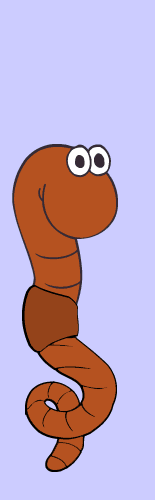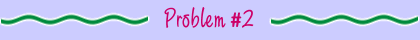Correct! There are 4 cubic feet in a bin that is 8 inches deep x 2 feet wide x 3 feet long. First, you multiply the depth (8 inches) by the width (24 inches) by the length (36 inches) to get 6,912 cubic inches. Then you divide the number of cubic inches by 1,728 (the number of cubic inches in a cubic foot) to get the number of cubic feet in the bin. 6,912 ÷ 1,728 = 4 cubic feet